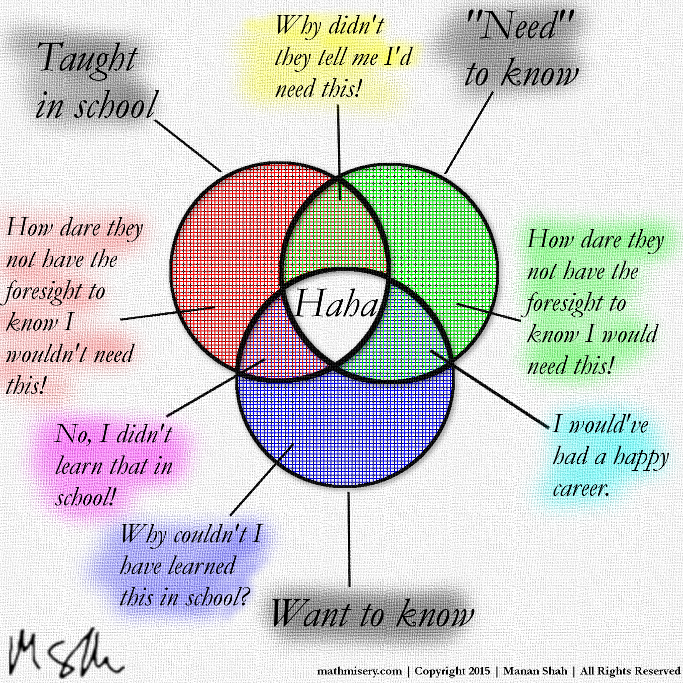## Daily Challenge:

Slow Version:

Fast Version:

Follow Task 1: Why does the first function work? How is it called the first time? Can it give different values at different values of `n`?

Speed Task 2: Why does the first version go slower than the second version?

# Class 12: Iterators

Today we're going to go under the hood of Python a little bit more than normal. I want to show you how Python sees loops.

To begin we're going to make extra sure that you know all of the basic container/sequence/iterable types that you can iterate through. These are our basics, including two that are new for you:

The Sequences:

• `list` `[1, 2, 3]`
• `range` `range(100, 0, -3)`
• `tuple` `(1, 5, 7)`
• `str` `"Andy rules"`

The collections:

• `dict` `{1: 'a', 2: 'b'}`
• `set` `{4, 2, 7}`

IO (input/output) streams:

• a file `open('andy.txt')`

What all of these have in common is that they can be turned into iterators, which allows loops to walk through them (and some other cool super powers).

Our goal today is to look at these iterables (and even make our own) then look at actions we can do on any iterable. That way you'll get a quadratic amount of power today.

## Sequences

Sequence types are ways of holding data where the placement in the structure matters. A list orders its entries and is made for ever changing, ordered, data (think: end of day stock prices for the last year). A tuple is an immutable structured data holder (think: `(x,y)` as the coordinates of a point). While range is a simple sequence generator (think: count by 7s from 400 to 900). Of course `str`, strings, are ordered arrangements of characters (which you've used to submit essays since you were young).

The sequence operations can be found at Python 3's documentation page. Here are some highlights, (s,t are sequences; i,j,k indices, n an integer, v a value)

• v in s
• s*n
• s[i:j:k]
• len(s)
• min(s)
• max(s)
• s.count(v)
• s + t
• s[i:j:k] = t (t some other iterable)
• del s[i:j:k]
• s.append(v)
• s.extend(t)
• s.reverse()
• s.insert(i, v)

Huge Tuple Task 3: Create a tuple `t = (9, 5, 8, 4, 7, 3, 6, 2, 5, 1)`, and make another tuple `s = (20, 30, 40)`. Try each of the operations above on a tuple (and use the other to extend or even add them). Which ones don't work? Why?

Range Play Task 4: Create a range `r = range(100, 0, -3)`. What does `r[5:33:3]` return? What error do you get when you try `r[5:33:3] = range(10)`?

List Play Task 5: Now do `L = list(r)` followed by `L[5:33:3] = range(10)`, what does `L` have now? For fun try `L[:2] = range(7)` and examine `L`.

String Play Task 6: Make a one-line statement which gives every third character in the string `"Andy is the greatest"`.

## Collections

These are unordered ways of collecting data without repeating keys. A `dict` stores both a `key` and a `value`, while a `set` only stores `key`s. The `set` is just like the mathematical version of a set with unions, intersections, and difference.(How to complain about school)

Venn Diagram Task 7: Use Python to help build the Venn diagram for `twos, threes` and `fives`.

## File IO

This topic could be a whole lecture unto itself, so we'll be light on the details. You can have Python read a file, line by line, or all at once. Then you can use it like you would any string.

Cloud9 Task 8: Go to cloud9 and setup a new workspace. Create a file named `myfile` and copy If by Rudyard Kipling into the file. Then open a python terminal (bash and type `ipython3` ). Type `f = open('myfile')`. Now do ```for line in f:```, `print(line)` inside the loop. Count the number of times the word `make` appears in the poem.

You can also write to a file, which we'll talk about in a future lecture.

## Iterating

So all of those types that we played with have one thing in common. They can be passed to the function `iter(t)`. "Who cares" you say? I do! This is how `for` loops work, and much more!

Here is the low-down: `it = iter([4,2,3,1])` makes an "iterator" out of that list. An iterator is an object which can be called with `next(it)`. That creates the next object, and eventually throws a specific type of error (more on that later too).

So a "for loop" could be recreated by calling next until you get an error, like this:

It gets cooler. These functions tend to work on iterables:

• sum
• len
• min
• max
• sorted
• set
• list
• tuple
• dict
• enumerate
• zip
• filter
• map
• reversed

There are also some very cool tools in `itertools` the library.

Sorted Task 9: Adapt the above example to print the characters of "Andy rulz", sorted.

Comprehensions: are ways of building iterables from other iterables.Squared Evens Task 10: Use list comprehension and range to generate a list of the even perfect squares less than 1000.

## Daily Challenge

Figure out what `zip` does. Use it to create a randomized scatter plot.